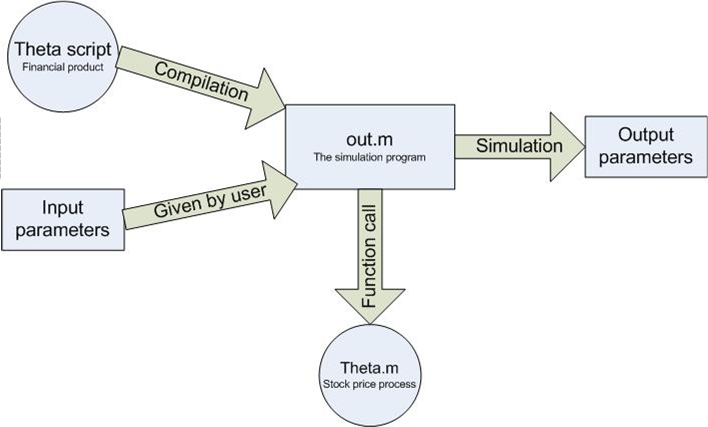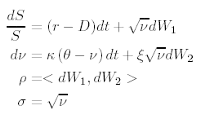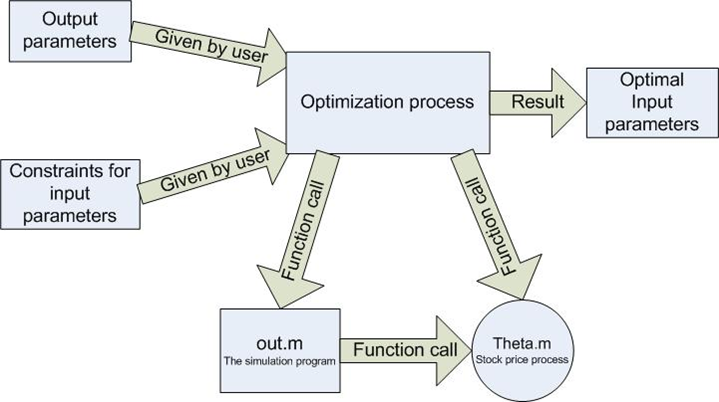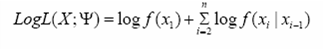# The Theta (financial) Fitter

Deepak Pandey, Janos Benk and Fayssal El Moufatich did their honours project colaboration with the Thetaris company.Figure 1: Pricing Concept and Heston Model.

The project was done in colaboration with the Thetaris company, who developed a new fast pricing concept of financial instruments.Figure 2

The pricing concept (see Figure 1 left) is based on script modeling of the financial instrument. This Theta script describes the cashflows in the contract and combined. This script combined with one underlying model (like Heston, see Figure 1 right) can be easily priced with fast Monte-Carlo methods.

The goal of the project was to solve the invers problem, to find the input variables which generate the given output of the Monte-Carlo simulation (see Figure 2). The solution of this invers problem poses several chalenges. The first challenge is to state the objective function in general way (see Figure 3).Figure 3: The Maximum Liklihood Objectiv Function.

We categorized the inverse problem in 3 different classes and build for them the objective functions. The second challenge was to use and implement methods which work in high dimensions. Since the program was implemented in Matlab we could use the Matlab optimization toolbox. Beside the Matlab optimization we implemented a sparse grid searching method which prooved to be an efficient heuristic search algorithm.